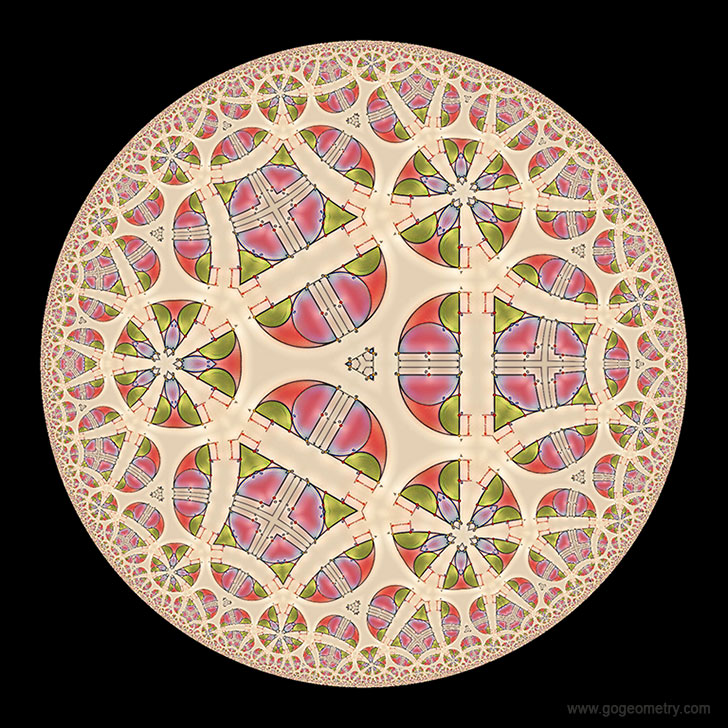Problem 284: Circular Sector 90 degrees, Semicircles, Tangent, Radius

The figure shows a circular sector AOB of 90 degrees with radius R. OB is the diameter of semicircle C. Semicircle D is tangent to semicircle C and arc AB at E and A, respectively. If r is the radius of the semicircle D, prove that r = R / 3.Dynamic Geometry: Geogebra of problem 284

GoGeometry Action 172! Author:Tim Brzezinski

Geometric Art: Hyperbolic Kaleidoscope of Problem 284 using iPad Apps